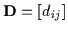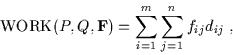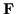# The Earth Mover's Distance

The Earth Mover's Distance (EMD) is a method to evaluate dissimilarity between two multi-dimensional distributions in some feature space where a distance measure between single features, which we call the ground distance is given. The EMD lifts'' this distance from individual features to full distributions.

Intuitively, given two distributions, one can be seen as a mass of earth properly spread in space, the other as a collection of holes in that same space. Then, the EMD measures the least amount of work needed to fill the holes with earth. Here, a unit of work corresponds to transporting a unit of earth by a unit of ground distance.

A distribution can be represented by a set of clusters where each cluster is represented by its mean (or mode), and by the fraction of the distribution that belongs to that cluster. We call such a representation the signature of the distribution. The two signatures can have different sizes, for example, simple distributions have shorter signatures than complex ones.

Computing the EMD is based on a solution to the well-known transportation problem . Suppose that several suppliers, each with a given amount of goods, are required to supply several consumers, each with a given limited capacity. For each supplier-consumer pair, the cost of transporting a single unit of goods is given. The transportation problem is then to find a least-expensive flow of goods from the suppliers to the consumers that satisfies the consumers' demand. Matching signatures can be naturally cast as a transportation problem by defining one signature as the supplier and the other as the consumer, and by setting the cost for a supplier-consumer pair to equal the ground distance between an element in the first signature and an element in the second. Intuitively, the solution is then the minimum amount of work'' required to transform one signature into the other.

This can be formalized as the following linear programming problem: Letbe the first signature with m clusters, where pi is the cluster representative and wpi is the weight of the cluster;the second signature with n clusters; andthe ground distance matrix where dij is the ground distance between clusters pi and qj.

We want to find a flow, with fij the flow between pi and qj, that minimizes the overall costsubject to the following constraints:The first constraint allows moving supplies'' from P to Q and not vice versa. The next two constraints limits the amount of supplies that can be sent by the clusters in P to their weights, and the clusters in Q to receive no more supplies than their weights; and the last constraint forces to move the maximum amount of supplies possible. We call this amount the total flow. Once the transportation problem is solved, and we have found the optimal flow, the earth mover's distance is defined as the work normalized by the total flow:The normalization factor is introduced in order to avoid favoring smaller signatures in the case of partial matching.

The EMD has the following advantages

• Naturally extends the notion of a distance between single elements to that of a distance between sets, or distributions, of elements.

• Can be applied to the more general variable-size signatures, which subsume histograms. Signatures are more compact, and the cost of moving earth'' reflects the notion of nearness properly, without the quantization problems of most other measures.

• Allows for partial matches in a very natural way. This is important, for instance, for image retrieval and in order to deal with occlusions and clutter.

• Is a true metric if the ground distance is metric and if the total weights of two signatures are equal. This allows endowing image spaces with a metric structure.

• Is bounded from below by the distance between the centers of mass of the two signatures when the ground distance is induced by a norm. Using this lower bound in retrieval systems significantly reduced the number of EMD computations.

• Matches perceptual similarity better than other measures, when the ground distance is perceptually meaningful. This was shown by  for color- and texture-based image retrieval.

More details on the EMD can be found in .

1
F. L. Hitchcock.
The distribution of a product from several sources to numerous localities.
J. Math. Phys., 20:224-230, 1941.

2
Y. Rubner, C. Tomasi, and L. J. Guibas.
A metric for distributions with applications to image databases.
In IEEE International Conference on Computer Vision, pages 59-66, January 1998.